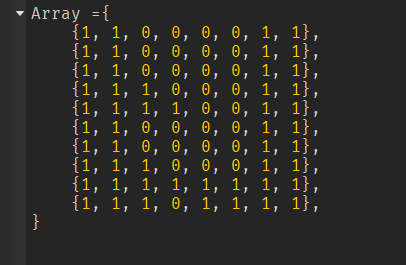# Making a more compact/pixelated 2D array

I’m trying to figure out how to turn an array like this:Into an array like this:I basically want it to become more pixelated. Can someone please explain to me how I would do something like this?

1 Like

To compact an array into a smaller one in Lua, you can use a loop or the table.pack function to create a new array that only includes non-empty or non-nil elements.

``````local originalArray = {1, 2, 3, "", nil, 5}
local compactArray = {}

for i, element in ipairs(originalArray) do
if element then
compactArray[#compactArray + 1] = element
end
end

print(unpack(compactArray))  -- Output: 1    2    3    5
--Here is an example with a loop
``````

However, you can also use the table.pack() function, which would look like this

``````local originalArray = {1, 2, 3, "", nil, 5}
local compactArray = table.pack(unpack(originalArray, 1, #originalArray))

print(unpack(compactArray))  -- Output: 1    2    3    5
``````

In both examples, the compactArray variable will contain a new array with only the non-empty or non-nil elements from the originalArray.

Btw, no idea is roblox is past LUA 5.2, if it is then this would work.

Since you’re scaling down by an integer (2 in this case), it’s fairly simple. Each sample in the scaled down version is made from the corresponding 2x2 square of samples in the original. There’s a few ways you can turn a 2x2 square into a single value, e.g. taking the average, min or max. It looks like you want the max, so any 2x2 square with at least one 1 turns into a 1 in the scaled down version.

Hmm, I ended up some code that does what you asked, here it is:

``````function image_dimensions(image)
--Personally I'd set image.w and image.h in image_new instead of counting every time
return #image, #image
end

function image_get(image, x, y)
local w, h = image_dimensions(image)
if x <= w and y <= h then
return image[y][x], true
end
return nil, false
end

function image_set(image, x, y, value)
local w, h = image_dimensions(image)
if x <= w and y <= h then
image[y][x] = value
return true
end
return false
end

function image_new(w, h, default)
local image = table.create(h)
--image.w=w; image.h=h; -- How I'd do it, see image_dimensions
for y = 1, h do
image[y] = table.create(w, default)
end
return image
end

function image_iter(image)
local w, h = image_dimensions(image)
local y, x = 1, 0
return function()
x = x + 1
if x > w then
y = y + 1
x = 1
end
if x <= w and y <= h then
return x, y
end
end
end

function image_max(image)
local result
for ix, iy in image_iter(image) do
local sample = image_get(image, ix, iy)
if sample then
if result then
result = math.max(result, sample)
else
result = sample
end
end
end
return result
end

function image_scale_down(image, factor, f_kernel_to_sample)
local wo, ho = image_dimensions(image) --Old dimensions
local wn, hn = math.ceil(wo/factor), math.ceil(ho/factor) --New dimensions
local result = image_new(wn, hn, -1) -- -1 indicates invalid value

local kernel = image_new(factor, factor, -1) -- -1 indicates invalid value
--Foreach sample in new, scaled down image
for xn, yn in image_iter(result) do
--Upper left corner of (factor*factor)- square of samples in old image
local yo = ((yn - 1) * factor) + 1
local xo = ((xn - 1) * factor) + 1

--Foreach sample in said square of samples in old image
--print("XN", xn, "YN", yn)
for xk, yk in image_iter(kernel) do
--Store image sample in the kernel, to be processed
local yi, xi = yo+yk-1, xo+xk-1
local sample, in_bounds = image_get(image, xi, yi)
if not in_bounds then sample = nil end
image_set(kernel, yk, xk, sample)
--print("S", sample, "xk,yk", xk, yk, "i", xi, yi)
end

image_set(result, xn, yn, f_kernel_to_sample(kernel))
end

return result
end

local some_image = { --7x4
{1, 1, 0, 0, 0, 0, 1},
{1, 1, 0, 0, 0, 0, 1},
{1, 1, 0, 0, 0, 0, 1},
{1, 1, 0, 0, 0, 0, 1},
}

local scaled = image_scale_down(some_image, 2, image_max) --4x2
print(image_dimensions(scaled))
for x, y in image_iter(scaled) do
local s = image_get(scaled, x, y)
print(s)
end
``````
2 Likes

This topic was automatically closed 14 days after the last reply. New replies are no longer allowed.